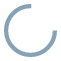### more

facts wiki news games kidztube appsThe factorial of a whole number n, written as n!, is found by multiplying n by all the whole numbers less than it. For example, the factorial of 4 is 24, because 4 × 3 × 2 × 1 = 24. Hence one can write 4! = 24. For some technical reasons, 0! is equal to 1.

Factorial can be used to find out how many possible ways there are to arrange n objects. view more...report a search problem